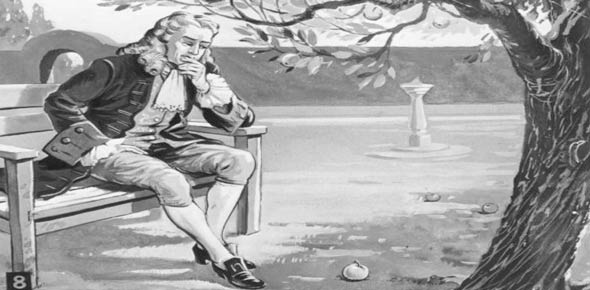# Newton's Laws Of Motion

4 Questions | Total Attempts: 150SettingsThis quiz will test your knowledge of Newton's Laws of motion, free body diagrams, and force problems

Related Topics
• 1.
Where does friction come from?
• A.

Applied force

• B.

Normal Force

• C.

Weight

• D.

Surfaces

• E.

None of the above

• 2.
The two forces that are related to each other by the coefficient of friction are
• A.

Weight and Normal force

• B.

Friction and Normal force

• C.

Friction and Weight

• D.

Applied force and Friction

• E.

Applied force and Normal force

• 3.
Which forces exist on the 2.2 kg mass in the following figure?
• A.

Weight and Normal force

• B.

Weight and Friction

• C.

Weight and Applied force

• D.

Applied force and friction

• E.

Weight

• 4.
If an object is moving at constant velocity, this means that
• A.

No net force acts on the object

• B.

It is accelerating

• C.

No force at all acts on the object

• D.

There is no friction

• E.

The object is moving to the right